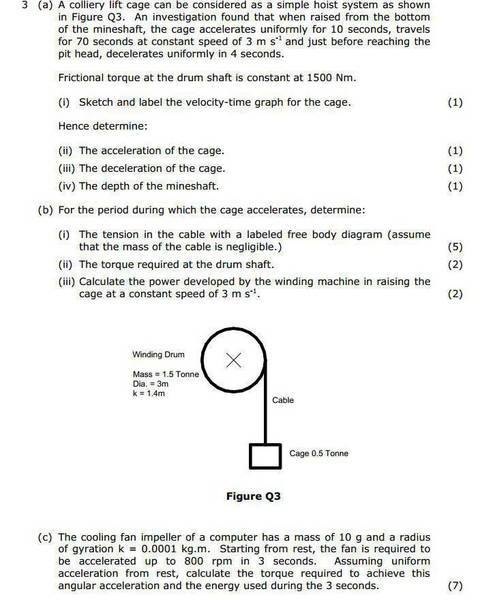# Having Trouble with (b) and (c) - A Physics Question

• smr101
In summary, the tension in the cable is 5055N and the torque required to provide that tension is 7582.5 + 1500 = 8171Nm.f

#### smr101

Hi, I'm having problems with (b) and (c) in this question. I've included the solution and part (a) for reference.http://snag.gy/x00Ou.jpg

I've worked out the distance for the acceleration area to be 15m which corresponds with the answers for (a).

I thought this would then give torque as 15 x 1500 =22500N. But that doesn't
seem to be the case.

Edit: I understand now that if I get the angular acceleration and sum of moments it's easy to work out the tension from that. But I'm unsure how to get the moments in order to then get the angular acceleration.

Getting thrown off by the diagram giving weight in 'tonnes' as well, no idea if that is relevant or not but I've always used kg.

Help is much appreciated, thanks!

Last edited:
I suggest a different approach. One that they hint in the question...

b(i) Asks for a free body diagram. If you do that I think you will find it easier to work out the tension.

Edit: I think it's even easier than that. I see in part a(ii) you worked out the acceleration of the cage. You know it's mass so what simple equation relates force, mass and acceleration?

b(ii)...Once you have the cable tension from b(I) then b(ii) will be a lot easier...
I thought this would then give torque as 15 x 1500 =22500N. But that doesn't seem to be the case.
No. I suggest you review your understanding of torque.

The relevant equation is...

Torque = force * distance

Where in this case..
"Force" is the tension you calculated in b(i).
"distance" is not the distance the cage moves. It's the radius of the drum.

Edit: Don't forget to add to that the frictional torque.

Last edited:
Torque = force * distance

Where in this case..
"Force" is the tension you calculated in b(i).
"distance" is not the distance the cage moves. It's the radius of the drum.

Edit: Don't forget to add to that the frictional torque.

Ok, thanks. So, I've got 1.5 x 1500 = 2250N for for frictional torque.

I've got 5055 x 1.5 for the toque = 7582.5N

Where have I gone wrong?

The problem says the friction torque is a constant 1500 NM. Note the units are Newton Meters so it's already a torque. eg no need to multiply by the radius.

So the total torque is 5055 + 1500 = 6555 NM

Edit: To that you need to add the torque required to accelerate the drum itself.

Last edited:
The problem says the friction torque is a constant 1500 NM. Note the units are Newton Meters so it's already a torque. eg no need to multiply by the radius.

So the total torque is 5055 + 1500 = 6555 NM

Edit: To that you need to add the torque required to accelerate the drum itself.

How is the torque to accelerate the drum itself calculated?

Also how does 5055N become 5055Nm?

How is the torque to accelerate the drum itself calculated?

Torque = moment of inertia * angular acceleration

Also how does 5055N become 5055Nm?

Sorry I made a mistake earlier (in post #4). The tension in the cable is given by

Tension = Mass * acceleration
= 500 (9.81+0.3)
= 5055N

The torque required to provide that tension is..

5055N * 1.5M = 7582.5NM (as you calculated)

Then..
Total torque = Torque due to tension in cable + frictional torque + torque to accelerate the drum
= 7582.5NM + 1500NM + torque to accelerate the drum

Torque = moment of inertia * angular acceleration

Sorry I made a mistake earlier (in post #4). The tension in the cable is given by

Tension = Mass * acceleration
= 500 (9.81+0.3)
= 5055N

The torque required to provide that tension is..

5055N * 1.5M = 7582.5NM (as you calculated)

Then..
Total torque = Torque due to tension in cable + frictional torque + torque to accelerate the drum
= 7582.5NM + 1500NM + torque to accelerate the drum

How is the angular acceleration calculated?

Also the solution states total torque is 8171NM, 7582.5 + 1500 is already 9082.5NM.

How is the angular acceleration calculated?

I have answered that on other thread. (From the velocity of the rope and the dimensions of the drum).

Also the solution states total torque is 8171NM, 7582.5 + 1500 is already 9082.5NM.

I can't see a mistake in our working. I'm wondering if the book answer is wrong.

I have answered that on other thread. (From the velocity of the rope and the dimensions of the drum).

I can't see a mistake in our working. I'm wondering if the book answer is wrong.

Ok, thank you.

I've been able to work it out...

The 5055 x 1.5 = 7582.5 part is our correct.

After that you work out the angular acceleration as you said, which is a/r = 0.2.

You times that by the inertia of I = mk^2 = 2940, so 2940 x 0.2 = 588.

Add the 588 to 7582.5 and you get the answer of 8171Nm.

The 1500Nm doesn't seem to come into it at all.

Thanks for your help by the way, I had an exam on this topic today and it went well, luckily I managed to avoid and massively difficult torque questions!

Add the 588 to 7582.5 and you get the answer of 8171Nm.
The 1500Nm doesn't seem to come into it at all.

I think that confirms the book answer is wrong because the 1500Nm should be included.

Will be interesting to see if they made the same omission when calculating the power in the next part of the problem.